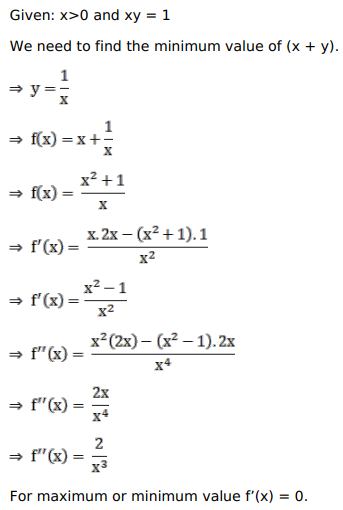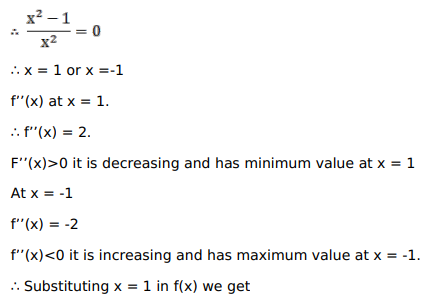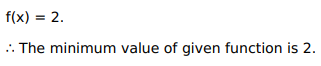# Solve this following

Question:

Mark $(\sqrt{)}$ against the correct answer in the following:

If $x>0$ and $x y=1$, the minimum value of $(x+y)$ is

A. $-2$

B. 1

C. 2

D. none of these

Solution: